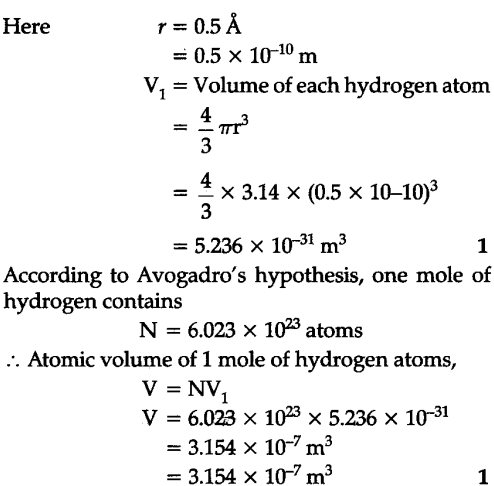# The unit of length convenient on the atomic scale is known as an angstrom and is denoted

The unit of length convenient on the atomic scale is known as an angstrom and is denoted by \$A\overset{0}{\mathop{{}}},\$. 1\$A\overset{0}{\mathop{{}}},\$ = \${{10}^{-10}}\$ m. The size of the hydrogen atom is about 0.5 \$A\overset{0}{\mathop{{}}},\$ . What is the total atomic volume in \${{m}^{3}}\$ of a mole of hydrogen atoms ?